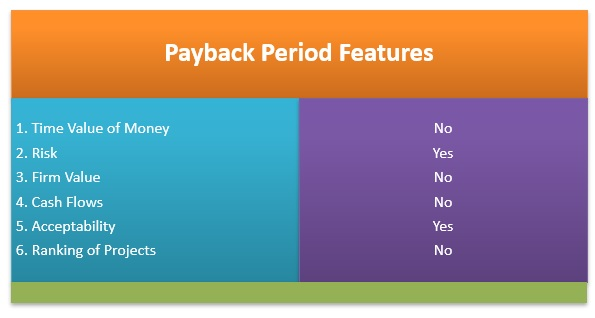# Payback Period

Posted in Finance, Accounting and Economics Terms, Total Reads: 2542

## Definition: Payback Period

Payback period calculates the financial return on investment based on the duration of time until the investment generates a return which is equal to the original amount of investment. Payback period is a method to evaluate the risks associated with a planned project and accordingly, accept or reject the project. Payback period doesn't consider time-value of money and is usually expressed in years. The payback period is a tool of analysis used in Capital Budgeting to decide whether a particular project should be taken or not or for choosing amongst two or more project opportunities.

It is defined as the time required to recover the investment made/ cost incurred in a project. Accordingly, a project with a shorter payback period is preferred over that with a longer one. It is often used in combination with other metrics of return on investment- the Net Present Value (NPV) and Internal Rate of Return (IRR).

## Importance of Payback Period

In this method, the project which promises the earliest recovery of the initial investment is considered more acceptable. If the actual payback period is shorter or equal to the management's required payback period, the project is accepted. For example, if a firm invests in equipment and wants to recoup the investment in 3 years, then if the project recovers the investment within 3 years, then that project is accepted. Fundamentally, the payback period method is used in the same way as a Breakeven Analysis, but unlike the latter, it considers the time span of return on investment instead of the number of sales units to cover the fixed costs. Often referred to as the "Back of the Envelope" due to its ease of calculation, this method is frequently used as an initial analysis which does not need much technical knowledge to understand and apply.## Formula for Payback Period

Mathematically, for a project with even cash inflows, it can be expressed as

Payback Period = Initial investment/ Annual Cash Inflows

In case of uneven cash inflows, payback period can be calculated as

Payback Period= n1+ CCFn1/CFn1+1

Where,
n1 is the no of years at which the last negative cumulative cash flow occurs;

CCFn1 is the absolute value of cumulative cash flow at the end of n1 years;
CFn1+1 is the total cash flow during the period following n1 years

Owing to its simplicity and ease of use, payback period is widely used in capital budgeting decision making. However, it has its limitations as it does not consider time value of money, risk, return and opportunity cost.

1. It is a very simple method which does not need prior knowledge to understand and use this method.

2. This method is useful for the firms for which liquidity is of utmost importance as any project with a short payback period can enhance the liquidity position of the business rapidly.

3. This method is useful to analyze how long the investment will be at risk. Hence, it gives a quick picture of the risk associated with a project.

4. It serves to be more useful in firms where investments tend to become obsolete very quickly and hence a quick return on investment is of grave concern.

5. Payback period is a useful capital budgeting method for cash-poor firms as a short payback period ensures fast inflow of cash.

1. The Payback period method does not take into account the time value of money (which means cash earned later worth lesser than current earnings). To eliminate this concern, a variation of this method, known as the Discounted Payback formula is used.

2. Though this method, discounts for risks with the projects, it is not necessary that two different projects get exposed to the same amount of risk in the same period. Usually, project risk is calculated by WACC (Weighted Average Cost of Capital).

3. While the payback method conveys the time required for the return on investment, it does not track the exact return on investment and hence the ultimate profitability of the project is not determined.

4. This method ignores the presence of additional cash flows that occur after the payback period.

5. This method does not take into account the asset lifespan if the useful life of the asset ends before or just after the payback period.

## Examples of Payback Period

XYZ Limited has two project options. Initial Investment for both the options is \$ 20,00,000.

Project A: Annual cash inflow of \$ 2,00,000

Project B: uneven cash flows

• Year 1 = \$3,00,000

• Year 2 = \$ 4,00,000

• Year 3 = \$ 6,00,000

• Year 4 = \$ 5,00,000

• Year 5 = \$ 6,00,000

Which project should be accepted?

For Project A:

Payback Period = Initial Investment / Annual cash inflow

Hence, Payback period is 10 years (20,00,000 / 2,00,000).

For Project B:

 Year Cash Flow (\$) Cumulative Cash Flow (\$) 0 -20,00,000 -20,00,000 1 3,00,000 -17,00,000 2 4,00,000 -13,00,000 3 6,00,000 -7,00,000 4 5,00,000 -2,00,000 5 6,00,000 4,00,000

The cumulative cash flow becomes positive in year 5. Hence the initial investment is recovered in between year 4 and year 5 i.e.; in between year 4 and year 5, \$ 2,00,000 is paid and the initial investment is recovered. So, it is :

2,00,000 / 6,00,000 = 0.33

Hence, the payback period is 4.33 years or 4 years and 4 months.

Since the payback period of project B is less, project B will be selected.

Hence, this concludes the definition of Payback Period along with its overview.

Browse the definition and meaning of more terms similar to Payback Period. The Management Dictionary covers over 7000 business concepts from 6 categories.

Search & Explore : Management Dictionary

Similar Definitions from same Category: